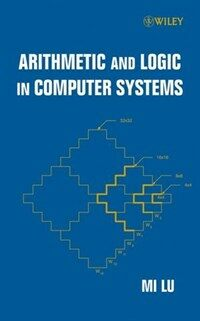> Detail View

# Detail View## Arithmetic and logic in computer systems (Loan 1 times)

Material type
단행본
Personal Author
Lu, Mi.
Title Statement
Arithmetic and logic in computer systems / Mi Lu.
Publication, Distribution, etc
Hoboken, NJ :   Wiley-Interscience ,   c2004.
Physical Medium
xxi, 246 p. : ill. ; 25 cm.
ISBN
0471469459 (cloth)
Bibliography, Etc. Note
Includes bibliographical references and index.
Computer arithmetic. Logic programming.
 000 00779camuu22002534a 4500 001 000045180507 005 20050712142403 008 031003s2004 njua b 001 0 eng 010 ▼a 2003062036 020 ▼a 0471469459 (cloth) 035 ▼a KRIC09506206 040 ▼a 211004 ▼d 211009 042 ▼a pcc 050 0 0 ▼a QA76.9.C62 ▼b L8 2004 082 0 0 ▼a 004/.01/51 ▼2 22 090 ▼a 004.0151 ▼b L926a 100 1 ▼a Lu, Mi. 245 1 0 ▼a Arithmetic and logic in computer systems / ▼c Mi Lu. 260 ▼a Hoboken, NJ : ▼b Wiley-Interscience , ▼c c2004. 300 ▼a xxi, 246 p. : ▼b ill. ; ▼c 25 cm. 504 ▼a Includes bibliographical references and index. 650 0 ▼a Computer arithmetic. 650 0 ▼a Logic programming.

### Holdings Information

No. Location Call Number Accession No. Availability Due Date Make a Reservation Service
No. 1 Location Call Number 004.0151 L926a Accession No. 111322945 Availability Available Due Date Make a Reservation Service

### Contents information

`Preface.  List of Figures. List of Tables. About the Author. 1. Computer Number Systems. 1.1 Conventional Radix Number System. 1.2 Conversion of Radix Numbers. 1.3 Representation of Signed Numbers. 1.3.1 Sign-Magnitude. 1.3.2 Diminished Radix Complement. 1.3.3 Radix Complement. 1.4. Signed-Digit Number System. 1.5 Floating-Point Number Representation. 1.5.1 Normalization. 1.5.2 Bias. 1.6 Residue Number System. 1.7 Logarithmic Number System. References. Problems. 2. Addition and Subtraction. 2.1 Single-Bit Adders. 2.1.1 Logical Devices. 2.1.2 Single-Bit Half-Adder and Full-Adders. 2.2 Negation. 2.2.1 Negation in One's Complement System. 2.2.2 Negation in Two's Complement System. 2.3 Subtraction through Addition. 2.4 Overflow. 2.5 Ripple Carry Adders. 2.5.1 Two's Complement Addition. 2.5.2 One's Complement Addition. 2.5.3 Sign-Magnitude Addition. References. Problems. 3. High-Speed Adder. 3.1 Conditional-Sum Addition. 3.2 Carry-Completion Sensing Addition. 3.3 Carry-Lookahead Addition (CLA). 3.3.1 Carry-Lookahead Adder. 3.3.2 Block Carry Lookahead Adder. 3.4 Carry-Save Adders (CSA). 3.5 Bit-Partitioned Multiple Addition. References. Problems. 4. Sequential Multiplication. 4.1 Add-and-Shift Approach. 4.2 Indirect Multiplication Schemes. 4.2.1 Unsigned Number Multiplication. 4.2.2 Sign-Magnitude Number Multiplication. 4.2.3 One's Complement Number Multiplication. 4.2.4 Two's Complement Number Multiplication. 4.3 Robertson's Signed Number Multiplication. 4.4 Recoding Technique. 4.4.1 Non-overlapped Multiple Bit Scanning. 4.4.2 Overlapped Multiple Bit Scanning. 4.4.3 Booth's Algorithm. 4.4.4 Canonical Multiplier Recoding. References. Problems. 5. Parallel Multiplication. 5.1 Wallace Trees. 5.2 Unsigned Array Multiplier. 5.3 Two's Complement Array Multiplier. 5.3.1 Baugh-Wooley Two's Complement Multiplier. 5.3.2 Pezaris Two's Complement Multipliers. 5.4 Modular Structure of Large Multiplier. 5.4.1 Modular Structure. 5.4.2 Additive Multiply Modules. 5.4.3 Programmable Multiply Modules. References. Problems. 6. Sequential Division. 6.1 Subtract-and-Shift Approach. 6.2 Binary Restoring Division. 6.3 Binary Non-Restoring Division. 6.4 High-Radix Division. 6.4.1 High-Radix Non-Restoring Division. 6.4.2 SRT Division. 6.4.3 Modified SRT Division. 6.4.4 Robertson's High-Radix Division. 6.5 Convergence Division. 6.5.1 Convergence Division Methodologies. 6.5.2 Divider Implementing Convergence Division Algorithm. 6.6 Division by Divisor Reciprocation. References. Problems. 7. Fast Array Dividers. 7.1 Restoring Cellular Array Divider. 7.2 Non-Restoring Cellular Array Divider. 7.3 Carry-Lookahead Cellular Array Divider. References. Problems. 8. Floating Point Operations. 8.1 Floating Point Addition/Subtraction. 8.2 Floating Point Multiplication. 8.3 Floating Point Division. 8.4 Rounding. 8.5 Extra Bits. References. Problems. 9. Residue Number Operations. 9.1 RNS Addition, Subtraction and Multiplication. 9.2 Number Comparison and Overflow Detection. 9.2.1 Unsigned Number Comparison. 9.2.2 Overflow Detection. 9.2.3 Signed Numbers and Their Properties. 9.2.4 Multiplicative Inverse and the Parity Table. 9.3 Division Algorithm. 9.3.1 Unsigned Number Division. 9.3.2 Signed Number Division. 9.3.3 Multiplicative Division Algorithm. References. Problems. 10. Operations through Logarithms. 10.1 Multiplication and Addition in Logarithmic Systems. 10.2 Addition and Subtraction in Logarithmic Systems. 10.3 Realizing the Approximation. References. Problems. 11. Signed-Digit Number Operations. 11.1 Characteristics of SD Numbers. 11.2 Totally Parallel Addition/Subtraction. 11.3 Required and Allowed Values. 11.4 Multiplication and Division. References. Problems.  Index.`

Information Provided By: :### New Arrivals Books in Related Fields

#### 실전 버그 바운티 : 웹 해킹 실무 가이드

Yaworski, Peter (2021)

#### 컴퓨터 구조 및 설계 : 하드웨어/소프트웨어 인터페이스

Patterson, David A (2021)

이효석 (2021)

김용태 (2021)

이광희 (2021)

김종원 (2020)

#### 디지털·미디어 리터러시 수업 : 블로그·팟캐스트·사진·인포그래픽·브이로그·영상·애니메이션·리믹스·소셜미디어 만들며 배우기

Hobbs, Renee (2021)

#### Cloud ethics : algorithms and the attributes of ourselves and others

Amoore, Louise (2020)

#### 비트의 세계 : 프로그래머의 눈으로 본 세상, 인간, 코드

Auerbach, David (2021)

김자미 (2021)

김자미 (2021)